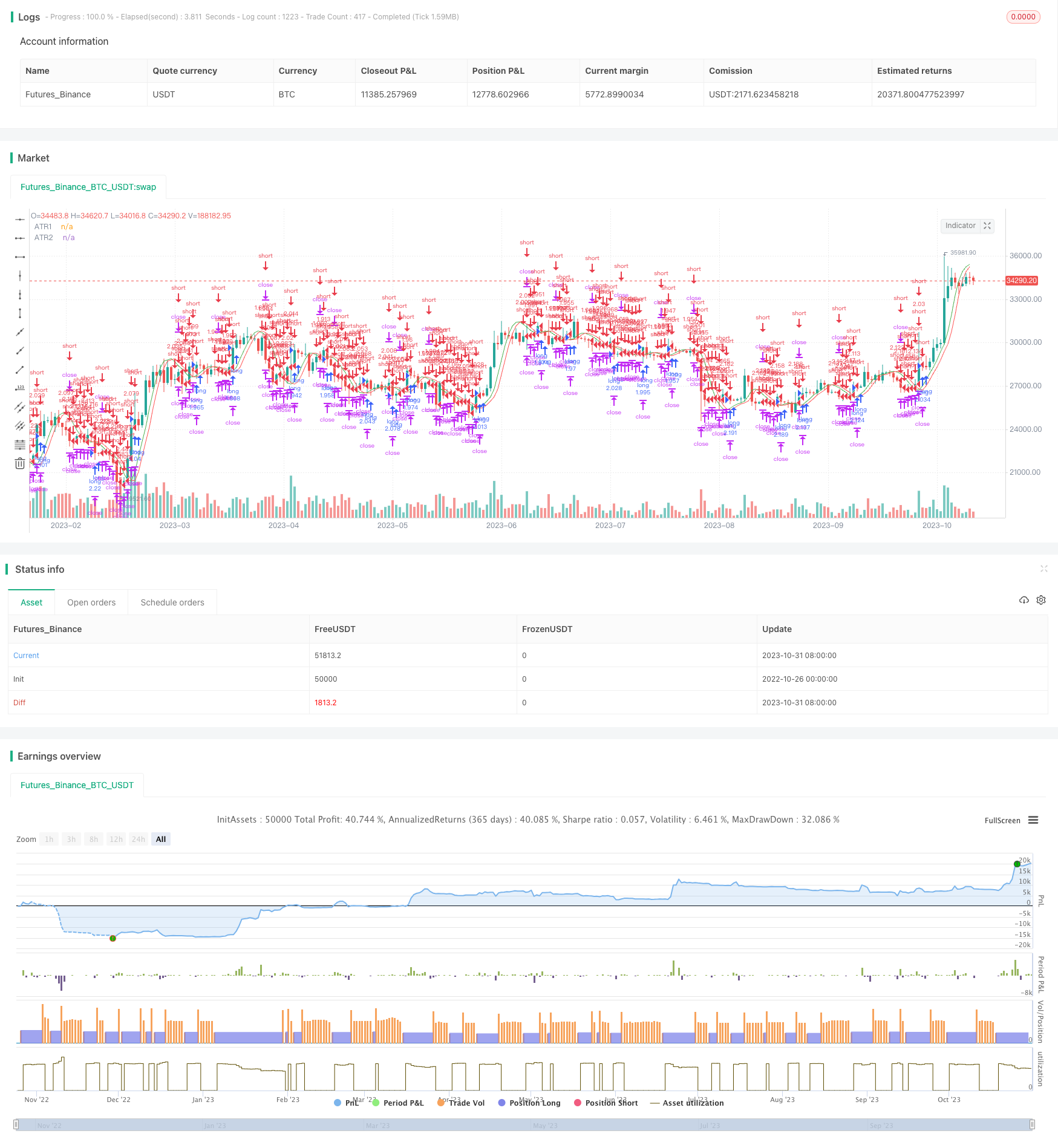Author: ChaoZhang, Date: 2023-11-02 16:51:14
Tags:## Overview

This strategy combines adaptive ATR moving average indicator and trend following for discovering trends in the market and trading along the trend. It uses Hull moving average to smooth ATR and form smooth ATR moving averages, then generates trading signals based on price’s relationship with the ATR moving averages. ATR moving averages can effectively filter market noise and identify significant trends. The strategy also sets fixed stop loss and take profit points to control risk/reward ratio per trade. Overall, this strategy aims to follow trends identified by adaptive ATR moving averages and achieve steady profit growth through strict risk management.

## Strategy Logic

The core indicator of this strategy is ATR moving average. ATR is an important volatility measurement tool, which measures market volatility and price fluctuations. ATR moving average is the smoothed ATR formed into a moving average line for comparison with price to determine trend.

Specifically, the strategy first calculates True Range, which is the difference between high and low prices of the day, and the maximum difference between previous close and current highest/lowest price. Then it applies Hull moving average method to smooth the TR and obtain adaptive ATR moving averages. The ATR moving averages can filter out high frequency market noise and only capture significant price swings.

After calculating the ATR moving averages, the strategy compares price with the ATR moving averages. When price crosses above the ATR moving average, it signals an upward trend, and the strategy goes long. When price crosses below the ATR moving average, it signals a downward trend, and the strategy goes short.

In addition, fixed stop loss and take profit ranges are set after each trade. When price hits the stop loss level, the trade is stopped out. When price hits the take profit level, profit is taken. This limits the loss and locks in profit for each trade.

In summary, this strategy combines adaptive ATR moving averages and strict risk management to follow significant trends and control loss per trade, in order to achieve steady profit growth.

The main advantages of this strategy are:

1. Using adaptive ATR moving averages to effectively identify significant trends and filter market noise to avoid being trapped.

2. Applying Hull moving average method to calculate smoother ATR moving averages, avoiding being misled by high frequency fluctuations.

3. Setting fixed stop loss and take profit to limit loss per trade and lock in profits, ensuring risk/reward ratio.

4. Trend following trading style can keep capturing trends and increase profit potential.

5. Simple and clear logic, easy to understand. Flexible parameter settings suit different products and markets.

6. Can be applied in any product for trend following. Highly adaptable.

## Risk Analysis

The main risks of this strategy are:

1. Possibility of false signals from ATR moving averages. Prices may fluctuate violently and cause errors in ATR moving average signals.

2. Stop loss being too tight increases chance of being stopped out. Ensure stop loss allows enough price movement.

3. Fixed take profit may exit too early, unable to capture full trends. Consider dynamic take profit based on ATR.

4. Sudden price spikes hitting stop loss. Need to pause trading during such events to prevent huge losses.

5. Failure to exit timely when trend reverses can lead to losses from reverse trend. Need to identify trend reversal signals.

6. Parameters need optimization for different products and markets. Otherwise it may affect strategy performance.

## Optimization Directions

The strategy can be optimized in the following aspects:

1. Optimize parameters of ATR moving average, including ATR period and smoothing parameters, which affect the ATR moving average.

2. Optimize stop loss and take profit strategy. Consider dynamic stops and targets based on ATR, instead of fixed values.

3. Add rules to determine trend reversal, combining other indicators, to avoid being trapped by reversals.

4. Test and optimize parameters for different products and market environments to find optimal parameters.

5. Add detection of extreme events, pause trading when huge price spikes occur to control loss.

6. Optimize entry timing, consider entering on pullbacks instead of breakouts to lower risk.

7. Parameter combination optimization, test different combinations of ATR period and smoothing parameters to find best match.

## Conclusion

In summary, this strategy uses adaptive ATR moving averages to identify trends, and trades the trends with fixed stop loss and take profit. ATR moving averages effectively identify trends, and fixed stops and targets control risk/reward. The advantages are simple and clear logic, easy to understand, adaptable to different products through parameter tuning. But risks include false signals, improper stop loss setting exist. Future improvements can be made through optimizing ATR moving average parameters, stop loss/take profit strategies, adding trend reversal detection etc. to further improve strategy performance.

```/*backtest
start: 2022-10-26 00:00:00
end: 2023-11-01 00:00:00
period: 1d
basePeriod: 1h
exchanges: [{"eid":"Futures_Binance","currency":"BTC_USDT"}]
*/

//@version=3
strategy("ATR(Hull)", overlay=true, default_qty_type=strategy.percent_of_equity, default_qty_value=100, calc_on_order_fills= false, calc_on_every_tick=true, pyramiding=0)
length = input(title="Length", defval=14, minval=1)
price = input(close)
SL = input(50, title="Stop loss")
TP = input(150, title="Take profit")
FromMonth = input(defval = 9, title = "From Month", minval = 1, maxval = 12)
FromDay = input(defval = 1, title = "From Day", minval = 1, maxval = 31)
FromYear = input(defval = 2018, title = "From Year", minval = 2017)
ToMonth = input(defval = 1, title = "To Month", minval = 1, maxval = 12)
ToDay = input(defval = 1, title = "To Day", minval = 1, maxval = 31)
ToYear = input(defval = 9999, title = "To Year", minval = 2017)
start = timestamp(FromYear, FromMonth, FromDay, 00, 00)
finish = timestamp(ToYear, ToMonth, ToDay, 23, 59)
window() => true
p=price
func_hma(style, length)=>
return = wma((2*wma(p,length/2))-wma(p,length),round(sqrt(length)))
ATR=func_hma(tr(true), length)
plot(ATR, title="ATR1",color=green,transp=0)
plot(ATR, title="ATR2",color=red,transp=0)
if (ATR>ATR)
strategy.entry("long",strategy.long,comment="Long",when=window())
if (ATR<ATR)
strategy.entry("short",strategy.short,comment="Short",when=window())
//strategy.close_all(when=strategy.openprofit<-eqSL and window())
//strategy.close_all(when=strategy.openprofit>eqTP and window())
strategy.exit("exit", "long", profit = TP, loss = SL)
strategy.exit("exit", "short", profit = TP, loss = SL)
```

More